## 3.4.1 WICK Theorem

The WICK decomposition allows a perturbation expansion of GREEN's functions. It always holds for zero-temperature GREEN's functions and only under the condition that field operators must be given in the interaction picture (Appendix B.2). Their time evolution is governed by the non-interacting HAMILTONian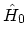, and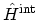is treated as a perturbation. If these conditions are fulfilled, WICK's theorem states that the expectation values of products of field operators is equal to the sum of expectation values of all possible pairs of operators and that each of these pairs will be a non-interacting single-particle GREEN's function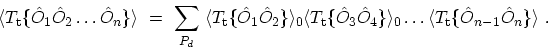(3.33)

The sum runs over all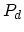distinct permutations of the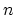indices. It should be noticed that brackets such as (3.33) vanish if the number of creation and annihilation operators is not the same. If the number of annihilated particles is not the same as the number of created particles, then the system will not come back to its ground-state. As a result the expectation value over the ground-state vanishes. With the same reasoning one concludes that if both operators appearing in a bracket are annihilation or creation operators, the expectation value disappears, otherwise one obtains an expression proportional to the non-interacting GREEN's function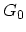.

The most general proof of this theorem is due to , where it is shown rigorously that the theorem holds exactly if the operators to be averaged are non-interacting and the density operator, which appears in the finite temperature formalism (see (3.15) and (3.26)), is a single-particle operator. Therefore, one can use the WICK theorem to get a perturbation expansion for the GREEN's function. The only formal difference from the equilibrium theory is the appearance of integration over a contour instead of integration over the inverse temperature interval for the case of finite temperature or the real axis for the case of zero temperature.

A few simple rules should be noted when making these pairings. The first is that a sign change occurs each time the positions of two neighboring FERMIon operators are interchanged.

The second rule concerns the time-ordering of combinations of operators representing different excitations. For example, a bracket with a mixture of electron and phonon operators can be separated into electron and phonon parts, since electron operators commute with phonon operators. WICK's theorem can be also applied to brackets of phonon operators. Since phonons are Bosons, sign does not change when exchanging positions of operators.

The third rule is a method of treating the time-ordering of two operators which are applied at the same time. The time-ordered product is undefined at equal times. To remove this ambiguity, the following interpretation can be applied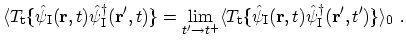(3.34)

WICK's theorem has been applied to calculate first-order perturbation expansions in Section 3.4.2. It can be also applied for higher-order perturbations.

M. Pourfath: Numerical Study of Quantum Transport in Carbon Nanotube-Based Transistors